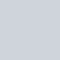# 先來看看今天的結果圖## -> 此篇文章的程式碼 github

Day14_分離合併RGB直方圖_histogram_split_merge.ipynb

# 運用 OpenCV 顯示圖片RGB直方圖、分離與合併RGB三通道

## 運用 OpenCV 分離RGB三通道

``````def split_RGBThreeChannel(img):
(B, G, R) = cv2.split(img) # 3 channel

# make all zeros channel
zeros = np.zeros(img.shape[:2], dtype = np.uint8)

print("R channel:")
show_img(merge_RGBThreeChannel(R=R, G=zeros, B=zeros))
print("G channel:")
show_img(merge_RGBThreeChannel(R=zeros, G=G, B=zeros))
print("B channel:")
show_img(merge_RGBThreeChannel(R=zeros, G=zeros, B=B))

return R, G, B
``````## 運用 OpenCV 合併RGB三通道

``````def merge_RGBThreeChannel(R, G, B):
img = cv2.merge([B, G, R])

return img
``````## 運用 OpenCV 顯示圖片RGB直方圖

``````def show_histogram(img):
# 畫出 RGB 三種顏色的分佈圖
color = ('b','g','r')
plt.style.use('dark_background')
plt.figure(figsize=(10,5))
for idx, color in enumerate(color):
histogram = cv2.calcHist([img],[idx],None,,[0, 256])
plt.plot(histogram, color = color)
plt.xlim([0, 256])

plt.show()
``````【沒錢ps,我用OpenCV!】Day 14 – 進階修圖1，運用 OpenCV 顯示圖片直方圖、分離與合併RGB通道 show histogram, split, merge RGB channel

# Reference

Python 與 OpenCV 加入線條圖案與文字教學

https://blog.csdn.net/sunny2038/article/details/9080047

https://www.jianshu.com/p/9fd339f806a7